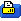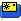Aug 14, 2019, 06:48 AM
"Come and take it B'yatch"
Help!

# Trifecta issues

Hello,

I need some help with some on the ground Tail servo jitter in Triflight. I have looked at other vids, and it seems to be a common issue. It could be a filter issue from what others have said. I do have the servo feed back wire, and done the tail tune, but need some suggestions. It oscillates slightly also, and need advice of where to start in the PID's. I do have screenshots of the PIDS as it sits, and will post them. Its running a Matek F405 Mini, and Triflight.

Here is a video of it on the ground, and flying.
Thanks

 Trifecta issues (5 min 12 sec)

# version
# Triflight on Betaflight 3.2.2 v2 / MATEKF405 (MKF4) 3.2.2 Dec 31 2017 / 15:24:22 (30f68c8) MSP API: 1.36

# master
set align_gyro = DEFAULT
set gyro_lpf = 42HZ
set gyro_sync_denom = 1
set gyro_lowpass_type = PT1
set gyro_lowpass_hz = 90
set gyro_notch1_hz = 400
set gyro_notch1_cutoff = 300
set gyro_notch2_hz = 200
set gyro_notch2_cutoff = 100
set moron_threshold = 48
set gyro_use_32khz = OFF
set align_acc = DEFAULT
set acc_hardware = AUTO
set acc_lpf_hz = 10
set acc_trim_pitch = 0
set acc_trim_roll = 0
set baro_bustype = I2C
set baro_spi_device = 0
set baro_i2c_device = 1
set baro_i2c_address = 0
set baro_hardware = AUTO
set baro_tab_size = 21
set baro_noise_lpf = 600
set baro_cf_vel = 985
set baro_cf_alt = 965
set mid_rc = 1500
set min_check = 1050
set max_check = 1900
set rssi_channel = 0
set rssi_scale = 41
set rssi_invert = OFF
set rc_interp = AUTO
set rc_interp_ch = RP
set rc_interp_int = 19
set fpv_mix_degrees = 0
set max_aux_channels = 14
set serialrx_provider = SBUS
set sbus_inversion = ON
set spektrum_sat_bind = 0
set spektrum_sat_bind_autoreset = ON
set airmode_start_throttle = 1350
set rx_min_usec = 885
set rx_max_usec = 2115
set serialrx_halfduplex = OFF
set input_filtering_mode = OFF
set blackbox_p_ratio = 32
set blackbox_device = SDCARD
set blackbox_on_motor_test = OFF
set blackbox_record_acc = ON
set min_throttle = 1070
set max_throttle = 2000
set min_command = 1000
set dshot_idle_value = 450
set use_unsynced_pwm = OFF
set motor_pwm_protocol = ONESHOT125
set motor_pwm_rate = 480
set motor_pwm_inversion = OFF
set thr_corr_value = 0
set thr_corr_angle = 800
set failsafe_delay = 4
set failsafe_off_delay = 10
set failsafe_throttle = 1000
set failsafe_kill_switch = OFF
set failsafe_throttle_low_delay = 100
set failsafe_procedure = DROP
set align_board_roll = 0
set align_board_pitch = 0
set align_board_yaw = 0
set gimbal_mode = NORMAL
set bat_capacity = 0
set vbat_max_cell_voltage = 43
set vbat_full_cell_voltage = 41
set vbat_min_cell_voltage = 33
set vbat_warning_cell_voltage = 35
set vbat_hysteresis = 1
set current_meter = ADC
set battery_meter = ADC
set vbat_detect_cell_voltage = 30
set use_vbat_alerts = ON
set use_cbat_alerts = OFF
set cbat_alert_percent = 10
set vbat_cutoff_percent = 100
set vbat_scale = 110
set ibata_scale = 400
set ibata_offset = 0
set ibatv_scale = 0
set ibatv_offset = 0
set beeper_inversion = ON
set beeper_od = OFF
set beeper_frequency = 0
set beeper_dshot_beacon_tone = 0
set yaw_motors_reversed = OFF
set 3d_deadband_low = 1406
set 3d_deadband_high = 1514
set 3d_neutral = 1460
set 3d_deadband_throttle = 50
set servo_center_pulse = 1500
set servo_pwm_rate = 300
set servo_lowpass_hz = 0
set tri_unarmed_servo = ON
set channel_forwarding_start = 4
set tri_unarmed_servo = OFF
set tri_tail_motor_thrustfactor = 54
set tri_tail_servo_speed = 274
set tri_servo_feedback = RSSI
set tri_motor_acc_yaw_correction = 0
set tri_motor_acceleration = 18
set tri_dynamic_yaw_boost = 240
set tri_servo_max_angle = 40
set reboot_character = 82
set serial_update_rate_hz = 100
set accxy_deadband = 40
set accz_deadband = 40
set acc_unarmedcal = ON
set imu_dcm_kp = 2500
set imu_dcm_ki = 0
set small_angle = 25
set auto_disarm_delay = 5
set disarm_kill_switch = ON
set gyro_cal_on_first_arm = OFF
set gps_provider = UBLOX
set gps_sbas_mode = WAAS
set gps_auto_config = ON
set gps_auto_baud = OFF
set gps_wp_radius = 200
set nav_controls_heading = ON
set nav_speed_min = 100
set nav_speed_max = 300
set nav_slew_rate = 30
set fixedwing_althold_reversed = OFF
set alt_hold_deadband = 40
set alt_hold_fast_change = ON
set deadband = 10
set yaw_deadband = 10
set yaw_control_reversed = OFF
set pid_process_denom = 1
set tlm_switch = OFF
set tlm_inverted = OFF
set tlm_halfduplex = ON
set frsky_default_lat = 0
set frsky_default_long = 0
set frsky_gps_format = 0
set frsky_unit = IMPERIAL
set frsky_vfas_precision = 0
set hott_alarm_int = 5
set pid_in_tlm = OFF
set report_cell_voltage = OFF
set ledstrip_visual_beeper = OFF
set sdcard_dma = OFF
set osd_units = IMPERIAL
set osd_rssi_alarm = 20
set osd_cap_alarm = 2200
set osd_alt_alarm = 100
set osd_ah_max_pit = 20
set osd_ah_max_rol = 40
set osd_tim1 = 2560
set osd_tim2 = 2561
set osd_vbat_pos = 2391
set osd_rssi_pos = 40
set osd_tim_1_pos = 54
set osd_tim_2_pos = 33
set osd_flymode_pos = 333
set osd_throttle_pos = 225
set osd_vtx_channel_pos = 377
set osd_crosshairs = 2248
set osd_ah_sbar = 200
set osd_ah_pos = 200
set osd_current_pos = 385
set osd_mah_drawn_pos = 353
set osd_craft_name_pos = 362
set osd_gps_speed_pos = 2263
set osd_gps_lon_pos = 82
set osd_gps_lat_pos = 65
set osd_gps_sats_pos = 2104
set osd_home_dir_pos = 2093
set osd_home_dist_pos = 2094
set osd_compass_bar_pos = 266
set osd_altitude_pos = 2295
set osd_pid_roll_pos = 423
set osd_pid_pitch_pos = 455
set osd_pid_yaw_pos = 487
set osd_debug_pos = 1
set osd_power_pos = 321
set osd_pidrate_profile_pos = 345
set osd_warnings_pos = 329
set osd_avg_cell_voltage_pos = 76
set osd_pit_ang_pos = 257
set osd_rol_ang_pos = 289
set osd_battery_usage_pos = 392
set osd_disarmed_pos = 138
set osd_nheading_pos = 311
set osd_nvario_pos = 2327
set osd_esc_tmp_pos = 82
set osd_esc_rpm_pos = 83
set osd_stat_max_spd = ON
set osd_stat_max_dist = OFF
set osd_stat_min_batt = OFF
set osd_stat_min_rssi = OFF
set osd_stat_max_curr = ON
set osd_stat_used_mah = ON
set osd_stat_max_alt = ON
set osd_stat_bbox = OFF
set osd_stat_endbatt = OFF
set osd_stat_bb_no = OFF
set osd_stat_tim_1 = OFF
set osd_stat_tim_2 = OFF
set task_statistics = ON
set debug_mode = TRI
set cpu_overclock = OFF
set pwr_on_arm_grace = 5
set vtx_halfduplex = ON
set vcd_video_system = 2
set vcd_h_offset = 0
set vcd_v_offset = 0
set max7456_clock = DEFAULT
set displayport_msp_col_adjust = 0
set displayport_msp_row_adjust = 0
set displayport_max7456_col_adjust = 0
set displayport_max7456_row_adjust = 0
set displayport_max7456_inv = OFF
set displayport_max7456_blk = 0
set displayport_max7456_wht = 2
set esc_sensor_halfduplex = OFF
set led_inversion = 0
set dashboard_i2c_bus = 1
set dashboard_i2c_addr = 60
set camera_control_mode = HARDWARE_PWM
set camera_control_ref_voltage = 330
set camera_control_key_delay = 180
set camera_control_internal_resistance = 470

# profile
profile 2

set dterm_lowpass_type = BIQUAD
set dterm_lowpass = 100
set dterm_notch_hz = 260
set dterm_notch_cutoff = 160
set vbat_pid_gain = OFF
set pid_at_min_throttle = ON
set anti_gravity_threshold = 350
set anti_gravity_gain = 1000
set setpoint_relax_ratio = 100
set dterm_setpoint_weight = 0
set acc_limit_yaw = 100
set acc_limit = 0
set crash_dthreshold = 50
set crash_gthreshold = 400
set crash_setpoint_threshold = 350
set crash_time = 500
set crash_delay = 0
set crash_recovery_angle = 10
set crash_recovery_rate = 100
set crash_limit_yaw = 200
set crash_recovery = OFF
set iterm_windup = 50
set iterm_limit = 150
set pidsum_limit = 500
set pidsum_limit_yaw = 400
set yaw_lowpass = 0
set p_pitch = 58
set i_pitch = 50
set d_pitch = 35
set p_roll = 40
set i_roll = 40
set d_roll = 30
set p_yaw = 70
set i_yaw = 45
set d_yaw = 20
set p_alt = 50
set i_alt = 0
set d_alt = 0
set p_level = 50
set i_level = 50
set d_level = 75
set p_vel = 55
set i_vel = 55
set d_vel = 75
set level_limit = 55
set horizon_tilt_effect = 75
set horizon_tilt_expert_mode = OFF
set gps_pos_p = 15
set gps_pos_i = 0
set gps_pos_d = 0
set gps_posr_p = 34
set gps_posr_i = 14
set gps_posr_d = 53
set gps_nav_p = 25
set gps_nav_i = 33
set gps_nav_d = 83

# rateprofile
rateprofile 0

set rc_rate = 100
set rc_rate_yaw = 100
set rc_expo = 0
set rc_expo_yaw = 0
set thr_mid = 50
set thr_expo = 0
set roll_srate = 70
set pitch_srate = 70
set yaw_srate = 70
set tpa_rate = 10
set tpa_breakpoint = 1650

# get master
Invalid name
Last edited by flashted; Aug 14, 2019 at 09:09 PM.
 Sign up now to remove ads between postsAug 14, 2019, 01:02 PM Registered User Tail jitter in Trifecta, a pandemic disease. Some medications: - Install a 1000mF capacitor directly in the PDB battery weldings. - Increase values for RC deadband and Yaw deadband. - Redo the endpoints servo calibration with eagle eye. - Install a good digital servo with metal gears. - Use teflon tape on the Trifecta yaw pinions to ensure a tight but free function. Whit this old glory you will have several hours of tuning PIDS. While quads calibration are very straightforward, tricopters (especially the plastic framed ones) need a very precise PIDs calibration you will only reach whit lot of trial and error.
 Aug 14, 2019, 09:16 PM "Come and take it B'yatch" Thanks for the suggestions I have already done most of what you have suggested. I forgot what the rating is on the cap though. I will increase values for RC deadband and Yaw deadband next Cheers Ted
 Aug 14, 2019, 09:21 PM "Come and take it B'yatch" PS the arms on this Trifecta have been modded with carbon fiber. 5mm cap strips along the outside of the front arms, although they are not visible. I used to do Tricopters with KK boards, but the Betaflight/Triflight stuff is a different animal.
 Aug 15, 2019, 04:46 PM USAF Vet Senior Member Just had jitter pop up on mine. Balanced all props and jitter is gone. Then the servo died! What a pain to change.
 Aug 15, 2019, 06:58 PM "Come and take it B'yatch" This is a jitter as it sits idle, in the tail servo. Yes big pain to change it. I have a digital in it now called a Dymond DD 150, with a feed back wire, and an emax w feedback wire waiting in the wings, but I am trying to get it to stop with the existing servo. It flys pretty good, but the jitter on the ground is an annoyance. I don't see David Windenstal's tri's doing it, do I know it can be figured.
 Aug 16, 2019, 04:34 PM USAF Vet Senior Member set tri_unarmed_servo = ON Set this to OFF and unarmed jitter will go!
 Aug 16, 2019, 10:06 PM "Come and take it B'yatch" Will do peterv, will try it tonight, and get back to you. t really is just an annoyance, but Im like that. I just don't like it when things are odd. Thank you
 Aug 16, 2019, 10:16 PM "Come and take it B'yatch" I think the uneven start in part could be a CG issue, although the motors do not all start together when I throttle up. I am running little bee esc's, with the later BL Heli firmware, and will try re calibrating everything again to see if it helps, but i've done this before to no avail. I am running a 2100 ma 3s lipo, which the instructions say is fine, and Quanum supplies a foam block to accommodate this.
 Aug 17, 2019, 09:48 AM USAF Vet Senior Member I run 1300ma 3s or 4s with 5 min flight times. 5s4 props 2 blade. It is a rocket on 4s.

 Thread ToolsShow Printable VersionEmail this Page